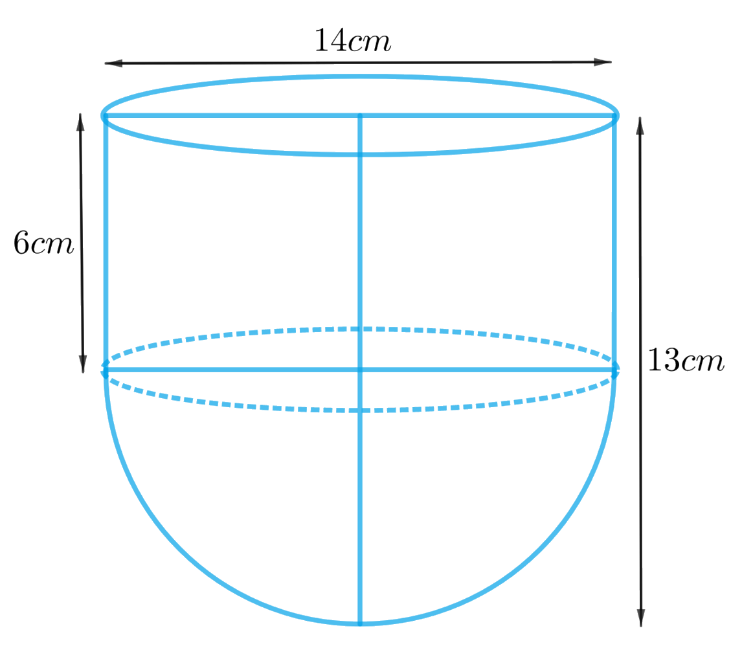# Ex.13.1 Q2 Surface Areas and Volumes Solution - NCERT Maths Class 10

Go back to  'Ex.13.1'

## Question

A vessel is in the form of a hollow hemisphere mounted by a hollow cylinder. The diameter of the hemisphere is $$14\;\rm{cm}$$ and the total height of the vessel is $$13 \;\rm{cm}$$. Find the inner surface area of the vessel.

## Text Solution

What is  known?

The diameter of the hemisphere is $$14\rm{ cm}$$ and total height of the vessel is $$13\rm {cm.}$$

What is unknown?

The inner surface area of the vessel.

Reasoning:

Create a figure of the vessel according to the given descriptionFrom the figure it’s clear that the inner surface area of the vessel includes the CSA of the hemisphere and the cylinder.

Inner surface area of the vessel $$=$$ CSA of the hemisphere $$+$$ CSA of the cylinder

We will find the area of the vessel by using formulae;

CSA of the hemisphere  $$= 2\pi {r^2}$$
where $$r$$ is the radius of the hemisphere

CSA of the cylinder $$= 2\pi rh$$
where $$r$$ and $$h$$ are the radius and height of the cylinder respectively.

Height of the cylinder $$=$$ Total height of the vessel $$–$$ height of the hemisphere

Steps:

Diameter of the hemisphere,$$d = 14 \rm cm$$
Radius of the hemisphere,  \begin{align}r = \frac{{14 \rm cm}}{2} = 7\rm{cm}\end{align}
Height of the hemisphere $$=$$ radius of the hemisphere, $$r = 7 \rm cm$$

Radius of the cylinder,$$r = 7 \rm cm$$

Height of the cylinder $$=$$ Total height of the vessel $$–$$ height of the hemisphere

$h = 13 \rm cm - 7cm = 6cm$

Inner surface area of the vessel $$=$$ CSA of the hemisphere $$+$$ CSA of the cylinder

\begin{align}&= 2\pi {r^2} + 2\pi rh\\&= 2\pi r\left( {r + h} \right)\\&= 2 \times \frac{{22}}{7} \times 7\rm{cm} \left( {7{cm} + 6{cm}} \right)\\&= 2 \times 22 \times 13 \rm {c{m^2}}\\&= 572 \rm {c{m^2}}\end{align}

Learn from the best math teachers and top your exams

• Live one on one classroom and doubt clearing
• Practice worksheets in and after class for conceptual clarity
• Personalized curriculum to keep up with school